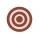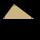# Quadratic equation + circle - math problems

#### Number of problems found: 29

• CircleFrom the equation of a circle: ? Calculate the coordinates of the center of the circle S[x0, y0] and radius of the circle r.
• Equation of circle 2Find the equation of a circle which touches the axis of y at a distance 4 from the origin and cuts off an intercept of length 6 on the axis x.
• Circle chordDetermine the radius of the circle in which the chord 6 cm away from the center of the circle is 12 cm longer than the radius of the circle.
• CircleCircle is given by centre on S[-7; 10] and maximum chord 13 long. How many intersect points have circle with the coordinate axes?
• TangentsTo circle with a radius of 41 cm from the point R guided two tangents. The distance of both points of contact is 16 cm. Calculate the distance from point R and circle centre.
• Circular flowerbedCircular flowerbed with diameter 8 m we split by concentric circle to circle and annulus with the same area. Find the radius of the circle.
• CircleWrite the equation of a circle that passes through the point [0,6] and touch the X-axis point [5,0]: ?
• RT and circlesSolve right triangle if the radius of inscribed circle is r=9 and radius of circumscribed circle is R=23.
• CircleThe circle touches two parallel lines p and q, and its center lies on line a, which is the secant of lines p and q. Write the equation of the circle and determine the coordinates of the center and radius. p: x-10 = 0 q: -x-19 = 0 a: 9x-4y+5 = 0
• Find parametersFind parameters of the circle in the plane - coordinates of center and radius: ?
• Circle and squareAn ABCD square with a side length of 100 mm is given. Calculate the radius of the circle that passes through the vertices B, C and the center of the side AD.Find the radius of the circle with area S = 200 cm².Find the radius of the circle using the Pythagorean theorem where a=9, b=r, c= 6+r
• Two chordsCalculate the length of chord AB and perpendicular chord BC to circle if AB is 4 cm from the center of the circle and BC 8 cm from the center of the circle.
• RT sidesFind the sides of a rectangular triangle if legs a + b = 17cm and the radius of the written circle ρ = 2cm.
• Right-angled triangleDetermine the content of a right triangle whose side lengths form successive members of an arithmetic progression and the radius of the circle described by the triangle is 5 cm.
• Ratio of sidesCalculate the area of a circle with the same circumference as the circumference of the rectangle inscribed with a circle with a radius of r 9 cm so that its sides are in ratio 2 to 7.
• Rhombus and inscribed circleIt is given a rhombus with side a = 6 cm and the radius of the inscribed circle r = 2 cm. Calculate the length of its two diagonals.
• Two chordsFrom the point on the circle with a diameter of 8 cm, two identical chords are led, which form an angle of 60°. Calculate the length of these chords.
• Distance problemA=(x, x) B=(1,4) Distance AB=√5, find x;

Do you have an interesting mathematical word problem that you can't solve it? Submit a math problem, and we can try to solve it.

We will send a solution to your e-mail address. Solved examples are also published here. Please enter the e-mail correctly and check whether you don't have a full mailbox.

Please do not submit problems from current active competitions such as Mathematical Olympiad, correspondence seminars etc...

Looking for help with calculating roots of a quadratic equation? Quadratic Equations Problems. Circle Problems.